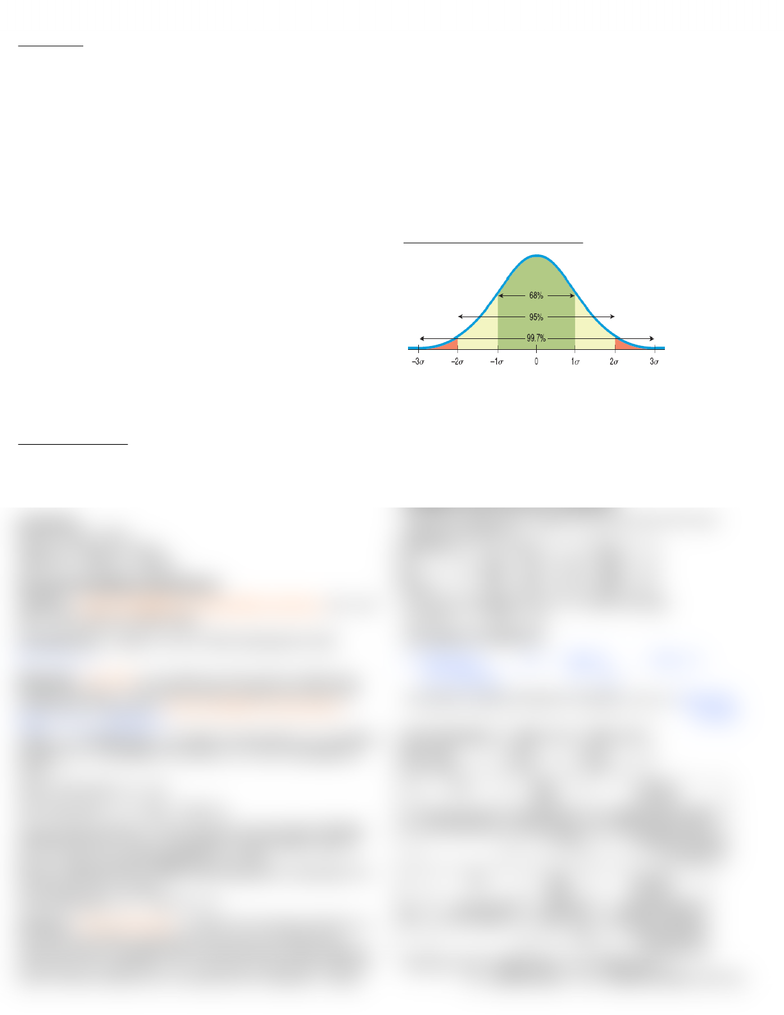# ADM 2303 Study Guide - Statistical Process Control, Percentile, Control Chart

103 views2 pages
School
Department
Course
ProfessorJaclyn Ebert 6221545
Probability
Marginal (straight): P(x)
P(x∩y) = P(x AND y) = P(x)*P(y)
P(xuy) = P(x OR y) = P(x) + P(y) – P(x AND y)
Mutually exclusive (disjoint): if the occurrence of one event
precludes the occurrence of another event.
Then P(x OR y) = P(x) + P(y)
Conditional: P(x|y) = P(x given that y has occurred)
=P(x AND y)/P(y) where P(y) > 0
Independent: when P(y|x) = P(y)
or P(x AND y) = P(x)*P(y)
vs. Disjoint: when P(x AND y) = 0 or when A and B overlap and
when both events can occur together.
Bayes Theorem:
I know P(x|y), and I want to know P(y|x).
I have an initial estimate of P(y), I do a test and find that x is true, so I
update my initial estimate to get P(y|x). Use tree diagrams, then
multiple along the branches to get P(x AND y). Then to find P(y|x), use
the conditional formula, and the denominator will be all the P(y)s
P(D|+) = P(+|D)*P(D) / [ P(+|D)*P(D) + P(+|W)*P(W) ]
Where: + = positive test result, D = patient has diabetes, W
= patient is well.
Random Variables
E(x) = EV = mean = μ = ∑x*P(x)
Var(x) = σ² = ∑(x – EV)²[P(x)] = ∑[x – x*P(x)]² [P(x)]
SD(x) = σ = √Var(x) = √∑[x – x*P(x)]² [P(x)]
Properties:
E(x+y) = E(x) + E(y)
Var(x+y) = Var(x) + Var(y)
SD(x+y) = √[Var(x) + Var(y)]
Discrete Probability Distrubutions
Uniform: equal probabilities for all possible outcomes. Ex: roll
dice. Use random variable table.
Combinations: Select x out of n with result good or bad.
C = n!/x!*(n – x!)
Binomial: x out of n, two possible outcomes with n identical and
independent trials. P of success remains constant from trial to trial.
Sampling with replacement. Known probability of being special.
P(x) = n!/x!*(n-x)!*(px)(qn-x)
where: n = sample size, x = number of successes, n-x = number
of failures, p = probability of success, q = (1-p) = probability of
failure.
Mean of binomial = μx = np
SD of binomial = σ = √npq = √np(1-p)
Normal Approximation: If np>10 and n(1-p)>10, then if P(≥50),
subtract 0.5 from x in the z calculation, and if P(≤50), add 0.5.
ex: μ = 10 for a normal distribution, σ = 2.83
P(10) = P(9.5≤x≤10.5) = P[(9.5-10)/2.83≤z≤(10.5-10)/2.83] = P(-
0.177≤z≤0.177) = 0.1405
Finite population: z = ( - μ)/ [σ√(N-n/N-1)]
Poisson: Known arrival rate. Average # of events per segment is λ.
# of events is random, occurrence of one does not influence the
chance an other. Probability that an event occurs in a given segment
is the same for all segments. Ex: # customers returning products per
month, # phone calls per min, # cracks per km of highway. Usually
time segments. Per – purr – cat – cat eats fish – poisson. Describes
the # of events in a given interval of time or space.
P(x) = ex)/x!
P(0 OR 1 OR 2 OR 3) = e(1/1 + λ1/1! + λ2/2! + λ3/3!)
P(>3) = 1 – P(0 OR 1 OR 2 OR 3)
Mean of poisson = μ = λ do rate*units… ex: rate 0.6, takes 1.7
hours, so 0.6*1.7=1.02 and that is your lamda
SD of poisson = σ = √λ
Exponential: Interval of time or space between events. Probability
density function.
f(x) = λe-λx where x ≥ 0
Standard Normal Distrubution
z = x-μ/σ where: x = any point on the horizontal axis, σ = SD of
the normal distribution, μ = population mean. Then use table.
Backwards: z = find P in middle of table somewhere, then:
x = μ + zσ
Random variables (RVs) ‘X’ and ‘Y’ represent annual incomes of well
established unmarried young professional men and women. These
two RVs are normally distributed and are statistically independent.
The mean values of ‘X’ and ‘Y’ are \$100K and \$80K with standard
deviations of \$20K and \$15K respectively.
Find P(The woman has a higher income than that of the man).
P(W>M) = P(W-M>0)
P(W-M>0) μ SD Var
W 80 15 ² => 225
M 100 20 ² => 400 +
W-M = -20 25 <= √ 625
z = (x-μ)/σ = (0+20)/25 = 0.8 P = 0.7881 from table
P(W>M) = 1 – 0.7881 = 0.21
Correlation Coefficient:
r = ∑(x-)(y-) sx = / ∑(x-)² = ∑xi / n
(n-1)(sx)(sy) √ n-1
Correlation between Random Variables: Corr(x,y) = ∑(x-)(y-)p
(sx)(sy)
Joint Probabilities Meter: \$1 Meter: \$2
Fine: \$0 0.5 0.4
Fine: \$50 0.01 0.09
x P(x) Mean
xP(x)
Variance
(x-)²P(x)
1 0.5+0.01=0.51 1(0.51)=0.51 (1-1.49)²(0.51)=0.12245
2 0.4+0.09=0.49 2(0.49)=0.98 (2-1.49)²(0.49)=0.1274
=1.49 Variance=0.2498
s=√Var=0.5
y P(y) Mean
yP(y)
Variance
(y-)²P(y)
0 0.5+0.4=0.9 0(0.9)=0 (0-5)²(0.9)=22.5
50 0.01+0.09=0.1 50(0.1)=5 (50-5)²(0.1)=202.5
=5 Variance=225
s=√Var=15
Corr(x,y) = [(1-1.49)(0-5)0.5 + (2-1.49)(0-5)0.4 +
(1-1.49)(50-5)0.01 + (2-1.49)(50-5)0.09] / (0.5*15)
x
µ
Unlock document

This preview shows half of the first page of the document.
Unlock all 2 pages and 3 million more documents.